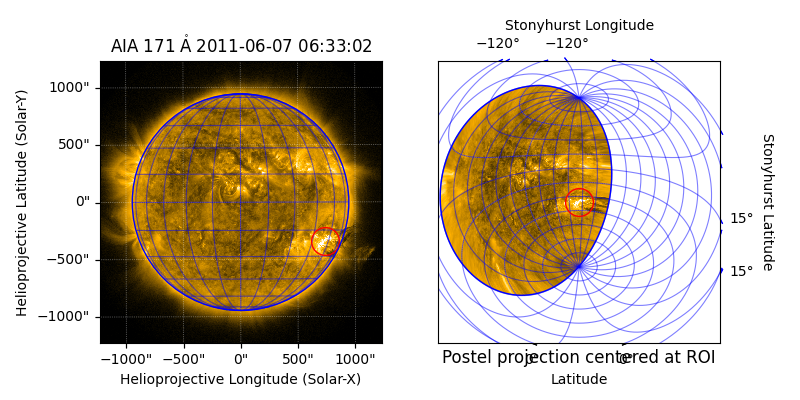# Reprojecting to a Map Projection with a Custom Origin#

In this example, we show how to reproject a map to a map projection with a custom origin. Here, we choose the target map projection to be the azimuthal equidistant projection, also known as the Postel projection, which has useful properties relative to a specified origin of the projection. If a different map projection is desired, modifying this example is straightforward.

import matplotlib.pyplot as plt

import astropy.units as u
from astropy.coordinates import SkyCoord

import sunpy.map
from sunpy.data.sample import AIA_171_IMAGE


We will use one of the AIA images from the sample data. We fix the range of values for the Map’s normalizer for a prettier image.

aia_map = sunpy.map.Map(AIA_171_IMAGE)
aia_map.plot_settings['norm'].vmin = 0
aia_map.plot_settings['norm'].vmax = 10000


Next, we create a SkyCoord to define the custom origin of the map projection. Here, we are going to center the projection at the helioprojective coordinates of a particular active region. We want our map projection to be in heliographic Stonyhurst coordinates, so we transform the origin coordinate accordingly.

origin_hpc = SkyCoord(735*u.arcsec, -340*u.arcsec, frame=aia_map.coordinate_frame)
origin = origin_hpc.heliographic_stonyhurst


We then create a FITS-WCS header that includes our custom origin coordinate. The azimuthal equidistant projection is specified by the code "ARC". See Supported projections for the projection codes for other projections.

out_shape = (750, 750)
out_shape,
origin,
scale=[0.4, 0.4]*u.deg/u.pix,
projection_code="ARC"
)


We reproject the map to our FITS-WCS header and copy over the plot settings.

out_map = aia_map.reproject_to(out_header)
out_map.plot_settings = aia_map.plot_settings


Finally, we plot both the original and reprojected maps side by side.

fig = plt.figure(figsize=(8, 4))


Plot the original AIA map, with the active region circled in red and the heliographic grid and solar limb in blue.

ax = fig.add_subplot(1, 2, 1, projection=aia_map)
aia_map.plot(axes=ax)
aia_map.draw_grid(axes=ax, color='blue')
aia_map.draw_limb(axes=ax, color='blue')
ax.plot_coord(origin, 'o', color='red', fillstyle='none', markersize=20)

[<matplotlib.lines.Line2D object at 0x7fb38d57f4f0>]


Plot the reprojected AIA map, again with the active region circled in red and the heliographic grid and solar limb in blue.

ax = fig.add_subplot(1, 2, 2, projection=out_map)
out_map.plot(axes=ax)
out_map.draw_grid(axes=ax, color='blue')
out_map.draw_limb(axes=ax, color='blue')
ax.plot_coord(origin, 'o', color='red', fillstyle='none', markersize=20)
ax.set_title('Postel projection centered at ROI', y=-0.1)
plt.show()/home/docs/checkouts/readthedocs.org/user_builds/sunpy/conda/latest/lib/python3.10/site-packages/sunpy/map/mapbase.py:2158: SunpyMetadataWarning: Missing metadata for observer: assuming Earth-based observer.
For frame 'heliographic_stonyhurst' the following metadata is missing: hglt_obs,dsun_obs,hgln_obs
For frame 'heliographic_carrington' the following metadata is missing: dsun_obs,crln_obs,crlt_obs

observer=self.observer_coordinate,


Total running time of the script: (0 minutes 1.661 seconds)

Gallery generated by Sphinx-Gallery